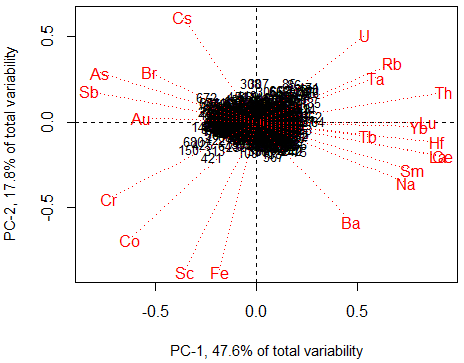Search
•Diana M. Benz, Ph.D.

# Understanding Geochemical Data in Mineral Exploration

Updated: Mar 1, 2019

When discovering a mineral resource, geochemistry is the foremost science used in determining the location and abundance of a potential deposit. Geochemistry, in mineral exploration, is the study of the relationships between the compositions of natural materials and geological processes. Geochemical data is typically defined in terms of quantities or ratios.Quantities are reserved for the amount of mineral resources or reserves within a defined area (a Mineral Resource Classification) and are measured in grams and kilograms or ounces and pounds. For example, a mineral resource may be estimated for grams or ounces of gold, silver or other measurements such as metal (e.g., gold) equivalent. These methods of measurement are only used during resource classification where the quality and quantity of samples collected are sufficient for determining the occurrence of the material of economic interest.

A ratio is the connection between two quantities and is often displayed as percent (%), parts per million (ppm), grams per tonne (g/t or gpt) and parts per billion (ppb). For example, 1 percent is one part within 100 parts and does not indicate a defined quantity. This method of measurement is primarily used during mineral exploration and when defining the grade (i.e., quality) of a mineral resource.

When researching the connection between chemistry and geology, ratio measurements (e.g. ppm) are typically used. Simple comparisons of chemical compositions with geological processes usually include displaying the ratios of one element on a map with bedrock units or geophysical properties. More complex associations include between element comparisons. Principal Component Analysis (PCA) is one method commonly used to determine multi-element associations.

{And now we get kind of 'sciency' ...}

Principal Component Analysis (PCA) was originally developed for large, complex data (> 50 samples) to determine the underlying relationships. The results are a set of 'scores' for a new set of simplified variables (graphically shown as a biplot) that, when interpreted, may be related to geological processes. In order to use PCA on geochemical data, i.e., ratios, the data should be transformed using a log-ratio method. This effectively 'opens up' the data so that each element can vary independently.

A biplot (shown below) is a graphical representation of the scores (black numbers) of two Principal Components (i.e., new simplified variables) plotted on x and y axes with the original elements (or variables) shown as vectors (red lines). Variables that plot closer together represent elements that behave coherently in a particular geological process.

Below are a series of biplots of Principal Component 1 versus 2 using Geological Survey of Canada regional stream sediment data RGS-37 instrumental neutron activation analysis (INAA) data processed by the log-ratio transformation/R-Q PCA (i.e., a correct method: R Statistics 'rgr' package gx.mva.closed algorithm) and various other transformation methods with R Statistics 'rgr' package gx.mva. All data was converted to ppm units (except where noted) and below detection limit values were imputed (except where noted) using the R Statistics robCompositions' impRZilr method.A Correct Method: Log-Ratio (clr) R-Q Principal Component Analysis of GSC regional stream sediment RGS-37 INAA data. Note the separation of the elements into coherent groups along the x-axis (47.6 % of the total variability) and the centre at 0,0. The clustering of the majority of the incompatible elements along the positive x-axis (x-axis = PC-1) are in close proximity to each other (REE: La, Ce, Sm, Yb, Lu & Tb; U, Rb, Ta, Th, Hf, and Ba). Along the negative x-axis (in inverse association with the incompatible elements) gold and its pathfinder elements are found: As, Sb, Au and Br (+/- Cs). The lower left quadrant represents the negative y-axis (y-axis = PC-2) showing incompatible elements with respect to mantle minerals.Incorrect Method: Raw data R-Q Principal Component Analysis of GSC regional stream sediment RGS-37 INAA data. An identical biplot was also produced with z-score normalized data. Note the poor separation of the elements compared to the log-ratio method and lack of strong inverse associations (upper quadrant heavy). This biplot exhibits a behavior commonly referred to as closure and will show up regardless of the number of elements used and/or their magnitude if an appropriate transformation is not performed.Incorrect Method: Log 10 transformation R-Q Principal Component Analysis of GSC regional stream sediment RGS-37 INAA data. Note the poor separation of the elements compared to the log-ratio method and the lack of strong inverse associations (upper quadrant heavy).Incorrect Method: Raw data with no conversion to ppm and below detection limit values replaced with half the detection limit values using R-Q Principal Component Analysis on GSC regional stream sediment RGS-37 INAA data. Note the poor separation of the elements compared to the log-ratio method and the lack of strong inverse associations (upper quadrant heavy).Incorrect Method: Raw data with no conversion to ppm and imputed values using R-Q Principal Component Analysis on GSC regional stream sediment RGS-37 INAA data. Note the poor separation of the elements compared to the log-ratio method and the lack of strong inverse associations (upper quadrant heavy). Also note the change in positions of all the elements when compared to the same raw data with half detection limit replacement values displayed above.

The interpretation of each of the biplots above would result in different assumptions regarding the geological processes involved in exploration for gold and could greatly impact future exploration planning. Geochemical data is typically the first data collected and the last data used to define a mineral deposit prior to mining. Understanding the chemistry and statistics behind the data is important for discovering new resources and for their development.